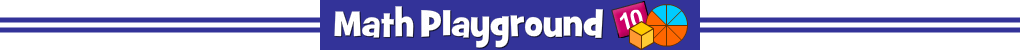Advertisement
Math Hoops (Flash)
Math Hoops - Learning Connections
Essential Skills
Mental Math - practice all four math operations
Problem Solving - read and understand word problems

Common Core Connection for Grades 3 and 4
Fluently multiply and divide within 100.
Use multiplication and division within 100 to solve word problems.
Solve two-step word problems using the four operations.
More Math Games to Play